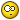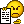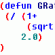# Need help with a Lisp Routine## Recommended PostsI was wondering what do i need to add or modify the attach file to produce a result like this " 1@length" right now it just produces "length". I'm a novice at lisp routines but i'm learning, i can change a few things to make it do what i need it to do but this ones has gotten me stumped. Any help would be greatly appreciated.Thanks,

Brian

```
;Extrusion Length
(defun c:EXTL  (/ cEnt tStr tBox tHgt tWid gr sPt cPt lAng bPt tPt pt1 pt2 pt3 pt4)
(if (and (setq cEnt (car (entsel "\nSelect Object: ")))
(member (cdr (assoc 0 (entget cEnt)))
'("LWPOLYLINE" "POLYLINE" "LINE")))
(progn
(setq tStr (rtos (- (vla-get-length
(vlax-ename->vla-object cEnt)) 4.))
tBox (textbox (list (cons 1 tStr) (cons 40 (getvar "TEXTSIZE"))))
twid (- (caadr tBox) (caar tBox)))
(princ "\nPosition Text...")
(while (eq 5 (car (setq gr (grread t 5 0))))
(redraw)
(if (listp (setq sPt (cadr gr)))
(progn
(setq cPt  (vlax-curve-getClosestPointto cEnt sPt)
lAng (angle cPt sPt)
bpt  (polar cPt lAng (/ (getvar "TEXTSIZE") 2.))
tpt  (polar bpt lAng tHgt)
mPt  (polar bPt lAng (/ tHgt 2.))
pt1  (polar bpt (+ lAng (/ pi 2.)) (/ tWid 2.))
pt2  (polar bPt (- lAng (/ pi 2.)) (/ tWid 2.))
pt3  (polar tpt (+ lAng (/ pi 2.)) (/ tWid 2.))
pt4  (polar tPt (- lAng (/ pi 2.)) (/ tWid 2.)))
(grvecs (list -3 pt1 pt2 pt3 pt4 pt1 pt3 pt2 pt4)))))
(if (eq 3 (car gr))
(progn
(setq lAng (- lAng (/ pi 2.)))
(cond ((and (> lAng (/ pi 2)) (<= lAng pi))
(setq lAng (- lAng pi)))
((and (> lAng pi) (<= lAng (/ (* 3 pi) 2)))
(setq lAng (+ lAng pi))))
(Make_Text mPt tStr lAng))))
(princ "\n<!> Incorrect Selection <!>"))
(redraw)
(princ))
(defun Make_Text  (pt val rot)
(entmake
(list
(cons 0 "TEXT")
(cons 8 (getvar "CLAYER"))
(cons 62 1)
(cons 10 pt)
(cons 40 (getvar "TEXTSIZE"))
(cons 1 val)
(cons 50 rot)
(cons 7 (getvar "TEXTSTYLE"))
(cons 71 0)
(cons 72 1)
(cons 73 2)
(cons 11 pt))))
```

Edited by BrianTFC
##### Share on other sitesPlease read the CODE POSTING GUIDELINES and edit your post.##### Share on other sitesDo you want just to add the "1@" prefix in front of the length? For this case, please modify the code like below.

```(setq tStr [color=blue](strcat "1@" [/color](rtos (- (vla-get-length
(vlax-ename->vla-object cEnt)) 4.0))[color=blue])[/color]
tBox (textbox (list (cons 1 tStr) (cons 40 (getvar "TEXTSIZE"))))
twid (- (caadr tBox) (caar tBox)))```

Other, I think you need to provide more details.

Regards,

Mircea

## Join the conversation

You can post now and register later. If you have an account, sign in now to post with your account.
Note: Your post will require moderator approval before it will be visible.×   Pasted as rich text.   Restore formatting

Only 75 emoji are allowed.